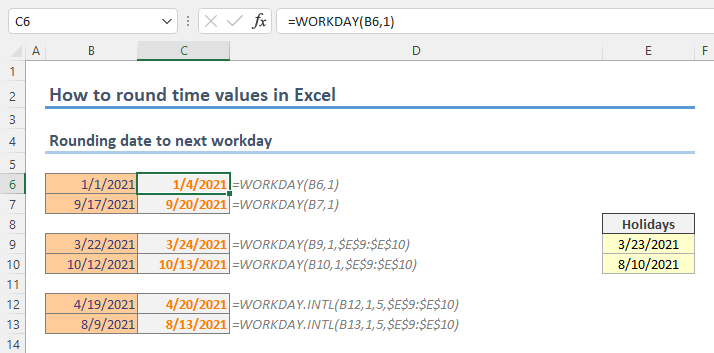Excel has extensive array of rounding functions as expected from a spreadsheet software. However, rounding date values is not as straightforward as rounding numeric values. In this guide, we’re going to show you how to round date values in Excel.

## Date and time concept in Excel

Let's say you want to round a date value to the nearest month. You cannot use the regular rounding functions like ROUND, ROUNDUP or ROUNDDOWN because of their decimal based limitation. You may think of using a 24 or 30-base numbers or multipliers with functions like MROUND, CEILING.MATH or FLOOR.MATH. Although the approach sounds rational, the results will be wrong thanks to calendar system's variety of concepts like weeks, months, or quarters.

On the other hand, you can use dates in calculations easily in Excel. Excel keeps date and time values as numbers. According to Excel, the history starts from Jan 1st, 1900 and accepts this date as 1. While whole numbers represent days, the decimal represents time. For example, while 1/1/2018 is equal to 43101, 12:00 is equal to 0.5. The date or time value you see in a worksheet is no more than a number formatting.

You can easily test this. Enter a date to a cell. You will see that the cell's number formatting is set to Date automatically. Converting the number format to General will show you the exact number.Unlike rounding time values, you cannot put your faith into single syntax. Each time period should be calculated by their dedicated functions.

## How to round date values

### Rounding date to next workday

Excel has dedicated functions that can return date values within workdays:

You can add or subtract a number of workdays from a given date by using each function. The WORKDAY.INTL function differentiate itself with a support of different weekend combinations.

WORKDAY(start_date, days, [holidays])WORKDAY.INTL(start_date, days, [weekend], [holidays])### Rounding date to previous or next specific weekday

To round a date value to specific weekday needs a simple math operation with the WEEKDAY function. The WEEKDAY function simply returns the day of the week of a given date in a specified day order.

WEEKDAY(serial_number, [return_type])

We use the option 2 in our model which enumerates the day from 1 to 7 by assuming 1 is Monday. So, the Sunday is 7.

You can use the following formula to calculate the next day. The last number indicates the day you want to get. Once again, 1 is Monday and 7 is Sunday.

=<date> - WEEKDAY( <date>, 2 ) + 7 + <day>The formula finds the first day of the current week by subtracting weekday from the date and adds 7 for the next week and finally the desired day's number to find the next specific weekday.

You can follow a similar approach to find the previous specific day by subtracting 7 instead of adding.

=<date> - WEEKDAY( <date>, 2 ) - 7 + <day>### Rounding date to nearest month

To round date to a month, DATE, DAY, MONTH and YEAR functions will be helpful. While the DATE function returns a date serial number by given year, month and day numbers, other functions simply return date parts resembling their names.

There is a simple logic behind to reach the nearest month. We can check if the day is greater than 15 or not. If it is, the formula will return the first day of the next week, otherwise the first day of the month the date within.

=DATE( YEAR( <date> ), MONTH( <date> ) + ( DAY( <date> ) > 15 + 0 ), DAY( 1 ) )The tricky part is the month parameter of the DATE function. We add the current month MONTH(B31) with a logical equation and a zero. Adding something with zero seems meaningless, however it allows us to convert the logical value to a numerical presentation. For Excel, TRUE equals to 1 and FALSE equals to 0. Using either value in a mathematical operation converts the logical value into a number.

Alternatively, you can use the N function or double unary (--) operator as well.

### Rounding date to next month

Our final tip is similar to the previous example. Since you can grab the month number by the MONTH function, all you need to do is to add 1 to that number and use the DATE function to return the desired date.